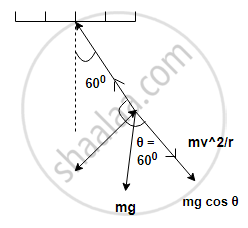Share

# A Small Body of Mass 0.3 Kg Oscillates in Vertical Plane with the Help of a String 0.5 M Long With a Constant Speed of 2 M/S - Physics

ConceptDefinition of Stress and Strain

#### Question

A small body of mass 0.3 kg oscillates in vertical plane with the help of a string 0.5 m long with a constant speed of 2 m/s. It makes an angle of 60° with the vertical. Calculate tension in the string (g = 9.8 m/s2).

#### SolutionGiven: m = 0.3 kg, r = l = 0.5 m, v = 2 m/s

To find: Tension (T)

Formulae: T_max=(mv^2)/r+mgcostheta

=(0.3xx4)/0.5+0.3xx9.8xxcos60^@

=3.87N

Is there an error in this question or solution?

#### APPEARS IN

2016-2017 (July) (with solutions)
Question 1.2 | 2.00 marks

#### Video TutorialsVIEW ALL 

Solution A Small Body of Mass 0.3 Kg Oscillates in Vertical Plane with the Help of a String 0.5 M Long With a Constant Speed of 2 M/S Concept: Definition of Stress and Strain.
S